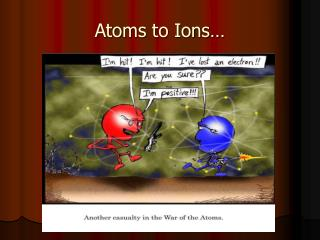DownloadDownload PresentationAtoms to Ions…

# Atoms to Ions…

Télécharger la présentation## Atoms to Ions…

- - - - - - - - - - - - - - - - - - - - - - - - - - - E N D - - - - - - - - - - - - - - - - - - - - - - - - - - -
##### Presentation Transcript

1. Atoms to Ions…

2. Have you noticed that some of the elements on your periodic table have more then 1 possible cation? • Vanadium, for example, has a 5+ and a 4+ cation. Cr, Fe, Cu also have two possible cations. • If you balance an equation that contains one of these elements, how will you know which cation was used?

3. The Classical System • Some elements are still referred to by their original Latin names, such as copper (cupric), tin (stannic), antimony (stibnic), lead (plumbic) etc. These names show the choice of charge by their ending…if the name ends in “ic” the larger charge is used. The “ous” ending means the smaller charge is used. This system is great if you know all the charges!

4. The elements that have Classical names are found on the Table of Ions. You will notice a Latin name and a “regular” name with Roman numerals. Use one or the other, not both. SnF4 = stannic fluoride (or tin (iv) fluoride) Fe2O3 = ferric oxide (or iron (iii) oxide) What is SbCl3?

5. The Stock System! • The Stock system was created by Alfred Stock in 1919. It is a simple and clear way of naming compounds which contain elements with several possible cations. • The choice of charge is stated in the name using Roman numerals.

6. How does it work? • When naming a binary compound, you use roman numerals after the cation to indicate the charge that was used. • (I) = 1 , (II) = 2, (III) = 3, (IV) = 4, (V) = 5 • Chromium (III) oxide  Cr2O3 • (III) indicates that the charge used is 3+ • If we were working with the 2+, the compound name would be chromium (II) oxide.

7. chromium(III) oxide • Now that we know that we are working with Cr3+, we can find its formula. • Cr3+ and O2- • Let’s “criss cross” the charges. • We are left with Cr2O3.

8. Find the formulas for: • Iron (III) oxide • Gold (III) chloride • Gold (I) sulfide

9. Finding the proper name… • Step 1 – Find the charge of the cation • We only have to find the charge of the cation if the element has more than one charge available. Ie. Mn, Cu, Co • Pb3P4 • How can we find the charge of Pb? • Do we know the charge of phosphide? • Yes we do!!!

10. The phosphide anion is 3- • There are 4 phosphide anions so the total charge is 12-  4 X 3- = 12- • This means that Pb3 is = 12+ because both sides have to balance!! • In Pb3, we have 3 atoms. • To find the charge of the Pb cation we divide the total charge by the number of atoms. 12 / 3 = 4

11. Pb3P4 • Each Pb atom has a charge of 4+ • The name of this compound is lead (IV) phosphide. • **If the compound has two subscripts, you can do a “reverse crisscross” and flip the subscripts back up.

12. Naming compounds with elements that have choice of charge. • 1. The cation (metal) is named first and the anion (non-metal) is named second. • 2. The second element is named by its anion name (ends in “ide”) • 3. Determine the charge of the cation and use roman numerals after the cation to state the charge.

13. Lets practice! • FeCl2 • TcO2 – what do we notice about Tc? *Note that some formulas have been reduced to the lowest common denominator…always check your total negative charges and assign the metal the opposite value. (ie 4- = 4+) • Cu3N